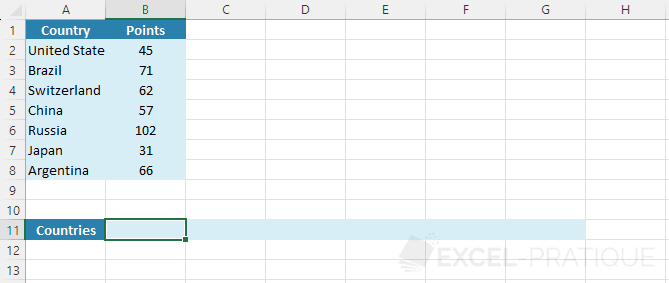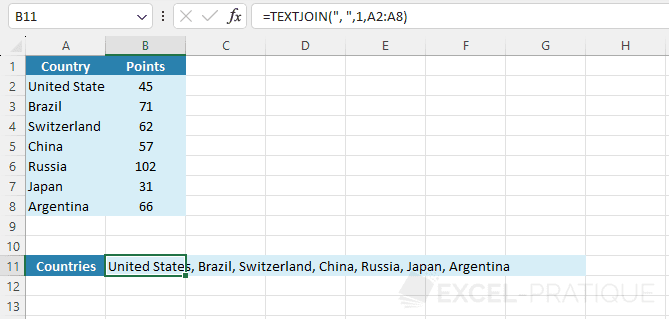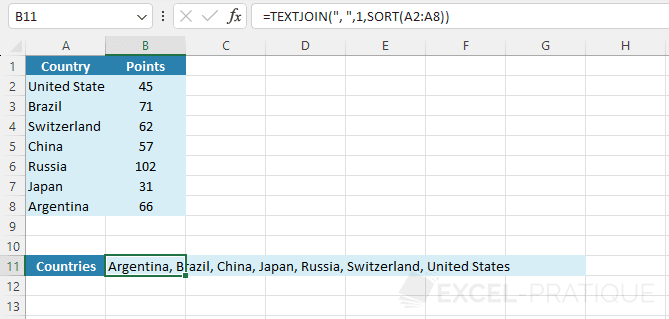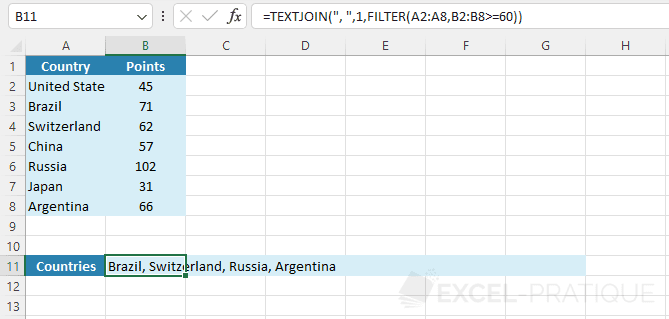# Excel Function: TEXTJOIN

The Excel function TEXTJOIN combines the values of one or more data ranges using the defined separator (and whether or not to include empty cells).

Usage:

`=TEXTJOIN(delimiter, ignore_empty, text)`

or

`=TEXTJOIN(delimiter, ignore_empty, text1, text2, ...)`

## Example of use

In this example, the goal is to get the list of all countries separated by , in cell B11:Select the TEXTJOIN function, enter the separator ", ", followed by the value 1 (or TRUE) to ignore empty cells (or 0 / FALSE to include them) and the range of country cells A2:A8:

``=TEXTJOIN(", ",1,A2:A8)``## Sort and join

To sort a range before joining its values, use the SORT function:

``=TEXTJOIN(", ",1,SORT(A2:A8))``## Filter and join

To filter a range before joining its values (for example to filter the countries that have scored at least 60 points), use the FILTER function:

``=TEXTJOIN(", ",1,FILTER(A2:A8,B2:B8>=60))``## Filter, sort and join

If necessary, you can combine the previous 2 examples to join filtered and sorted values:

``=TEXTJOIN(", ",1,SORT(FILTER(A2:A8,B2:B8>=60)))``